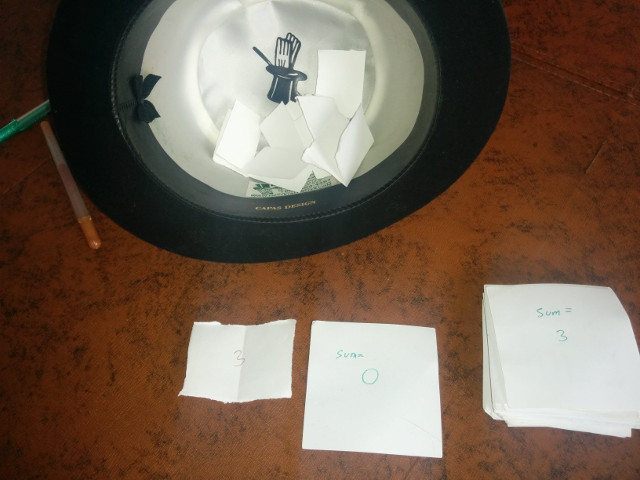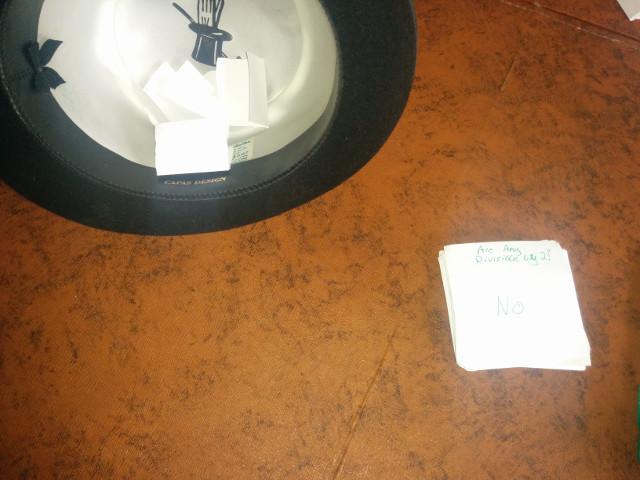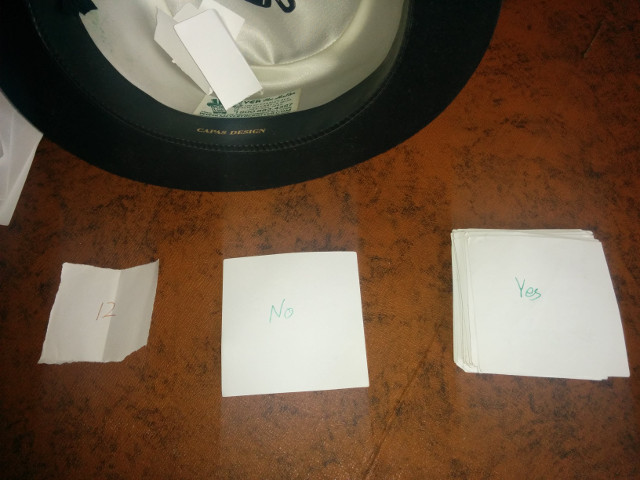# Learn reduce

So you’re a professional developer and you’ve heard of `Array.prototype.reduce` (or underscore/lodash’s `_.reduce` function), and you’ve heard that it’s an insanely powerful way of working with collections but you don’t quite get it.

tsk tsk tsk1

That’s ok. I’m here to help. It’s a more advanced concept that can be difficult to learn. Or it can be very easy.

Because you see, you already intuitively know how to do it. Let’s run through an exercise.

For this you will need

• A piece of paper
• A pen or pencil
• A stack of notecards or sticky notes
• A hat (the fancier the better)

To start with, take the piece of paper and tear it up into sixteen or so rectangular pieces. On each piece write some number. They don’t have to be all different but try not to follow any obvious pattern.Now fold each piece in half so that you can no longer see the number, shuffle them up, take about half of them and toss them in the hat.Set all the other pieces of paper to the side, put your stack of notecards or sticky notes in front of you, and let’s think of a question we can ask about the numbers in the hat.

We’ll start with the canonical `reduce` example: What is the sum of all these numbers?

We’re going to be pulling numbers from the hat one by one in order to answer the question. Two caveats: We’re not allowed to look at previous numbers, and we’re only allowed to remember one thing at a time. We will be using the cards as our “memory”.

Now let’s answer the question of the sum as best we can on the topmost card. We haven’t looked at any numbers yet so let’s start by saying the answer is zero.Great, now draw the first number and place the top card next to it so that the next blank card in the stack is showing. We have so far picked one number, and our previous sum is zero, therefore on the blank card write down the new sum.Now remove the number paper and the previous card, we won’t need them and are no longer allowed to look at them. Draw the next number and move the top card over near it as before. Again, given the previous sum, and this number, answer the question “what is the sum” on the next top card.Clear the current number paper and the previous card again and keep doing this until you’ve drawn the last number. There’s your answer!You’ve now answered the question by iteratively reducing the number of items in the hat.

In `reduce` function terms

• The number you drew is the item
• The “previous” card the accumulator
• The question you’re asking is the reducing function
• The top card is the reducing function result
• The initial card you wrote an answer on before you drew any numbers is the seed
• And the numbers in the hat to start is the collectionSince we now have the algorithm it should be dead simple to translate it. In javascript terms we have:

``````
var reducingFunction = function(accumulator, item) {
return accumulator + item;
}
collection.reduce(reducingFunction, 0);
``````

Or, if we follow the rule of naming things after what they do, rather than how they’re used, we get

``````
var sum = function(x, y) {
return x + y;
}
hatItems.reduce(sum, 0);
``````

Got it?

One more time for safetey?

Ok, new stack of cards, shuffle up the numbers, and toss some of them in the hat again.

This time, we’re going to answer the question “are any of the numbers divisible by two?”

As before, we will answer the question as best we can on the top card. Since we haven’t drawn any numbers yet, much less one that is divisible by two, we must answer “No”.Ok, let’s move the card over, draw the first number, and answer as best we can.We drew a 75, which is not divisible by two, and so far no numbers have been divisible by two so we write “No” again on the top card and draw the next number.This time, we drew a 21. Again, this is not divisible by 2 so yet again we write “No” and continue working our way through the hat.Now we drew a 12. That’s interesting since the answer to “is anything so far divisible by two” is now an emphatic yes, so that’s what we write down on the top card.

The next draw is also interesting.Even though we drew a one which is not divisible by two, we know that we previously did draw something that is divisible, so once again, the answer to our question of “is anything so far divisible by two” has to be yes!

In, fact, you might notice that now that we’ve answered yes, we can never answer no again. This makes sense since the question was if there is any number that is divisible by two. And of course, once we found a single one, the answer is absolutely. We still have to work through our example however, so let’s fast forward to the end.We have our answer. Translate it to code? Ok, one last time

``````
var areAnyDivisibleByTwo = function(areAnyPreviouslyDivisible, number) {
return areAnyPreviouslyDivisible || (number % 2 === 0)
}
hatItems.reduce(areAnyDivisibleByTwo, false);
``````

Think of some more questions, run through the exercise, and translate the algorithm to code and you too soon will be a reducing hero!

1 if you are just learning to program, you may remove up to two tsks